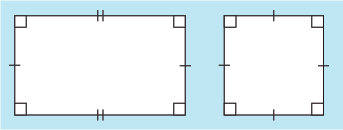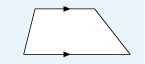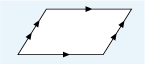Science, Maths & Technology

### Become an OU studentSucceed with maths: part 2

Start this free course now. Just create an account and sign in. Enrol and complete the course for a free statement of participation or digital badge if available.

# 1.3 Four-sided shapes

Shapes that have four straight edges are known collectively as quadrilaterals. The most familiar of these are squares and rectangles. It might seem very obvious how these are defined but a rectangle (it’s not called an oblong in maths!) has four straight sides that are all at right-angles to each other, with opposite pairs of lines being the same length. A square is a special kind of rectangle, where all the sides are the same length. This means that in both shapes, opposite pairs of lines are also parallel. These are both shown in Figure 7 – lines of the same length have been marked with a single or double short perpendicular line.Figure 7 A rectangle and a square

What if you have a four-sided shape where only one pair of lines is parallel? This is known as trapezium and an example is shown below in Figure 8. The parallel lines are marked with arrows.Figure 8 A trapezium

If the quadrilateral, a four-sided shape, has two sets of parallel sides, it is called a parallelogram:Figure 9 A parallelogram

Of course, this definition also means that squares and rectangles are parallelograms!

Now these more familiar shapes have been defined, it is useful to look at how to refer to specific sides or angles in a shape so that these can be clearly communicated to others. This is the subject of the next brief section.